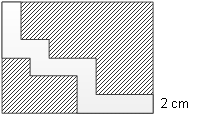### Sample Problem

Ben has a rectangular grassplot. It has 16 meters in length and 12 meters in width. A road of 2 meters in width goes through it, as shown. What is the area of the grassplot?

m2#### Solution

We see that we can shift the lower left plot of land up and to the right 2 meters such that it matches perfectly with the upper right plot of land. The combined plot of land is a new rectangle after removing the 2 meter wide road. We can work out the area of the grassplot by working out the length and the width of the new rectangle. The length of the new rectangle is 16 - 2 = 14 meters, and the width is 12 - 2 = 10 meters, so the area is 14 × 10 = 140 m2.